# Examples of Waveform Sampling

July 8, 2019

Typically, sampling involves taking measurements of a waveform at regular intervals and structuring these measurements as a sequence of values. The length of this interval is called the period and is often represented as 𝑇 or 𝜏 with the unit seconds. The rate 1/𝜏 is called the sample rate and is often denoted 𝖥s with the unit Hertz. Here is an example of sampling a cosine:

### Example 1: Sampling a Cosine

Suppose we have a waveform, w(t), that is a 100 Hz cosine such that w(t) = cos(2𝜋100t).

Sampling at 𝖥s = 4 x fh = 400 Hz (Figure 1.3) yields the sample sequence:

(1)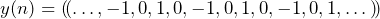Similarly, sampling at 𝖥s = 6 x fh = 600 Hz (Figure 1.3)  yields the following sequence:

(2)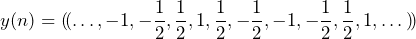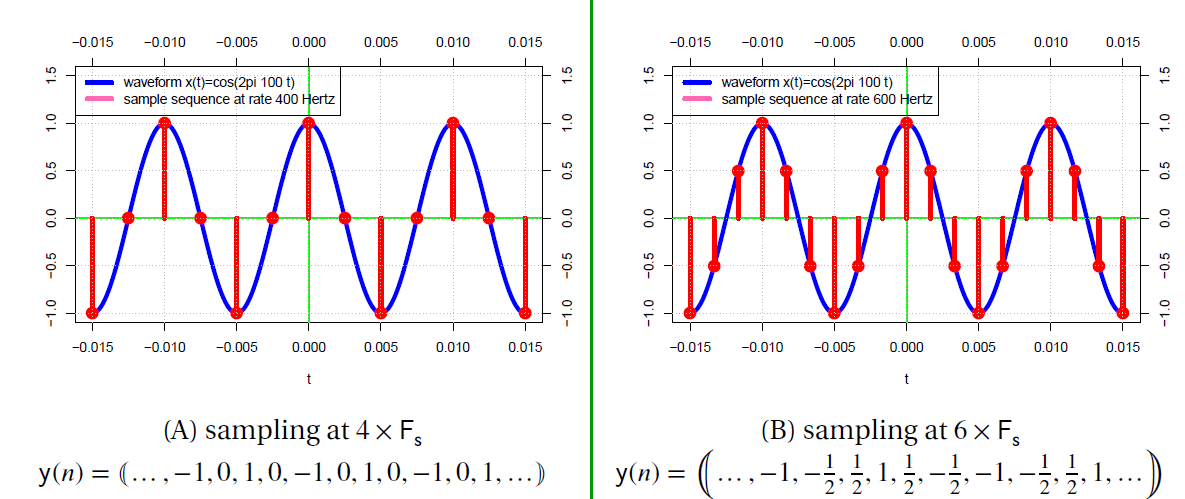Figure 1.3. Sampling a cosine.

Even though these two sequences are quite different, either can be used to perfectly reconstruct the original waveform w(t).

If a sampled signal is to be processed and eventually reconstructed, retaining the knowledge of the sample rate is extremely important. The concept of duration in time is lost without the knowledge of 𝖥s during sampling, even though the time-ordering of the samples is preserved (e.g. y(1) occurred earlier in time than y(2)). Our second example further illustrates this point.

### Example 2: Sampling a Cosine without the Sample Rate

Suppose we have a waveform w1(t) that is a 1 Hz cosine such that w1(t) ≜ cos(2𝜋t). Sampling at 4 Hz (Figure 1.4) yields the sequence:

(3)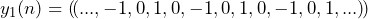Similarly, a waveform w2(t) that is a 100 Hz cosine such that w2(t) ≜ cos(2𝜋100t) sampled at 400 Hz (Figure 1.4) yields the sequence:

(4)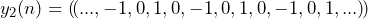The two sequences are identical (y1(n) = y2(n)) despite the fact that in real-time w1(t) is oscillating 100 times faster than w2(t).  Thus, we must know the value of 𝖥s to properly reconstruct the waveform in the real world.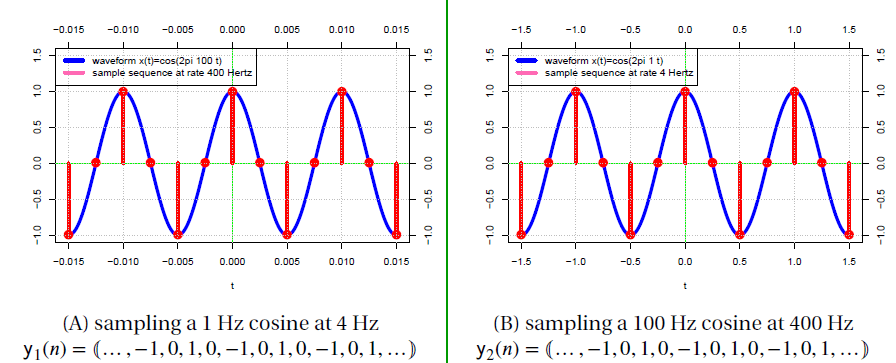Figure 1.4. Sampling a 1 Hz cosine at 4 Hz and a 100 Hz cosine at 400 Hz.